# 5G NR Cyclic Prefix (CP) Design

Cyclic prefix (CP) refers to the prefixing of a symbol, with a repetition of the end In OFDM wireless systems. The receiver is typically configured to discard the cyclic prefix samples A CP  can be used to counter the effects of multipath propagation. The basics of CP is available in following post.

What is Inter Symbol Interference in LTE, how Cyclic Prefix eliminate this ?

Multipath Signal Transmission

Radio channel between the base station and UE introduces delay spread in the time domain. This delay spread is generated by the transmitted signal reaching the receiver from multiple paths which have different distances environment, terrain, and clutter result in different delays.

Delay spread of the received signal pulse caused by multi-path is the difference between the maximum transmission latency in largest path and the minimum transmission latency in shorted path. The latency varies with the varies with the environment, terrain, and clutter, and does not have an absolute mapping relationship with the cell radius. This multi path delay spread can cause following:

• Inter-Symbol Interference (ISI), which severely affects the transmission quality of digital signals
• Inter-Channel Interference (ICI), the orthogonality of the subcarriers in the OFDM system is damaged, which affects the demodulation on the receive side

How Cyclic Prefix reducing ISI and ICI

• Guard Period: To avoid Inter Symbol Interference a guard period can be inserted between OFDM symbols in the form of Cyclic Prefix. This guard period provides a time window for the delay spread components belonging to the previous symbol to arrive before the start of the next symbol. The guard period could be a period of discontinuous transmission or could be a transmission of anything else. The length (Tg) of the guard period is generally greater than the maximum delay over the radio channel
• Cyclic Prefix: CP can be inserted in the guard interval to reduce ICI. Replicating a sampling point following each OFDM symbol to the front of the OFDM symbol. This ensures that the number of waveform periods included in a latency copy of the OFDM symbol is an integer in an FFT period, which guarantees sub carrier orthogonality. Copying the end of the payload and transmitting as the cyclic prefix ensures that there is a ‘circular’ convolution between the transmitted signal and the channel  response. This allows the receiver to apply a simple multiplication to capture the energy from all delayed components. If a ‘circular’ convolution was not completed then the receiver would experience ICI when completing the frequency domain multiplication

Key Factors to Determining CP Length

• Multi path Delay: The multiple and CP length is directly proportional. The larger the multipath delay, requires longer Cyclic Prefix
• Length of OFDM Symbol: Given the same OFDM symbol length, a longer CP can be  a large system overhead, so to control over overhead the length of CP shall be selected as appropriate.

The basic desing of CP in NR is similar to LTE and same overhead as that in LTE. CP design ensure that it aligned symbols between different SCS values and the reference numerology (15 kHz). For example, µ=15 khz a single slot have about 7 symbols resides in 0.5 mili seconds including the CPs for each symbols and µ=30 khz a single slot have about 14 symbols including CPs for each symbols within same 0.5 milli sec. So here the length of CP is adapted based on subcarrier spacing (fsc).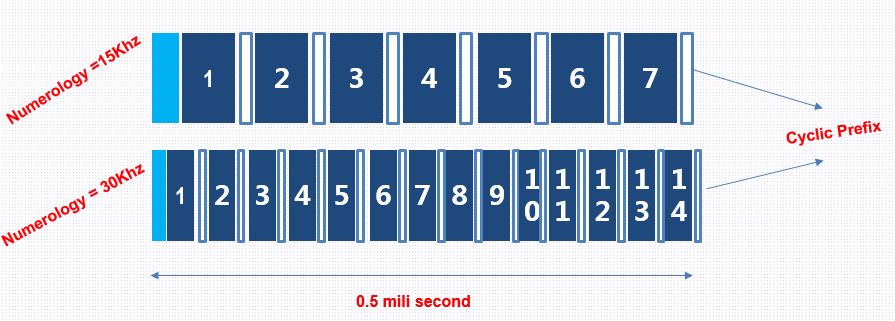Properties of CP in 5G NR

• 3GPP has specified two types of cCPs, Normal Cyclic Prefix (NCP) and Extended Cyclic Prefix (ECP).
• The NCP is specified for all subcarrier spacings
• ECP is currently only specified for the 60 kHz subcarricr spacing.
• If normal CP (NCP) is used, the CP of the first symbol present every 0.5 ms is longer than that of other symbols
• Cyclic prefix durations decrease as the subcarrier spacing increases

CP Length for Different Subcarriers

The CP length for different sub-carrier can be calculated using following formula.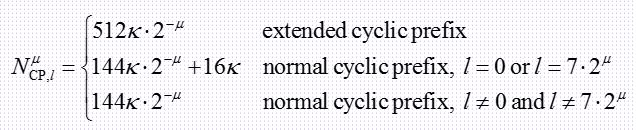and CP time duration can be using following formula.u is numerology, l is the symbol index here and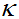is a constant  to relate NR basic time unit and LTE basic time unit and can be represented by following equation.Ts is LTE basic time unit and  Tc  is NR basic time unit. The details for timing can be read from following post.

5G NR Physical Layer Timing Unit

Below is the summary of Cyclic Prefix duration based on above formula. Each numerology has 2 long symbols per 1 ms sub frame. These longer symbols are generated by increasing the duration of the normal cyclic prefix, to ensure that each numerology has an integer number of symbols within each 0.5 ms time window, while also ensuring that as many symbol boundaries as possible coincide, e.g. every symbol boundary belonging to the 15 kHz subcarrier spacing coincides with every second symbol boundary belonging to the 30 kHz subcarrier spacing.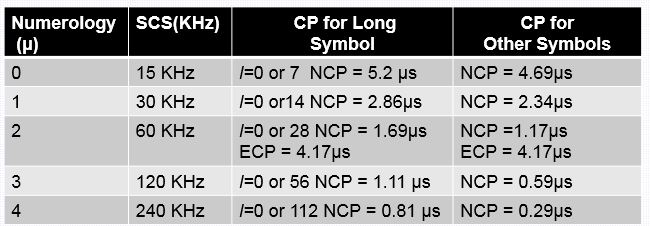The CP overhead is a percentage ratio of CP time duration and Symbol time duration, for example 15KHz the NR symbol duration is 66.67 μs and CP duration is 5.2 µs. Then overhead can be calculated 5.2/66.67 = 7.8 % . Here the long symbol shall have more overhead as CP where as other symbols shall have less overhead. below table provides a summary of overhead for Normal CP for different sub carrier spacing.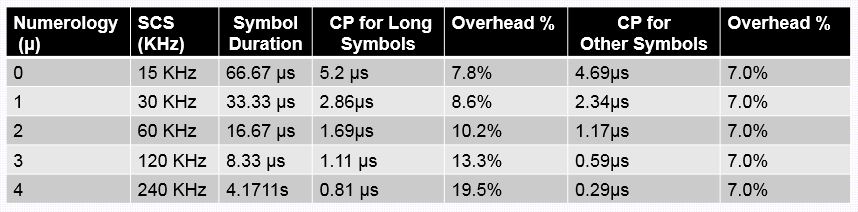Calculating Multi path support of each CP

The CP duration defines how much multiple distance is can support without affecting the Inter symbol interference (ISI) and Inter Carrier Interference (ICI). The distance be calculated using a simple Time, distance formula. For example, let’s take 15 KHz having CP for long symbol as 5.2 µs. The radio signal travel with velocity of light which is C= 3.0 x 108 m/s, then distance can be calculated as  velocity x time = (3.0 x 108 ) x (5.2 x 10-6 ) = 1560 meter. Similarly,  it calculated for other CPs and sub carrier spacing and summary is available in below table.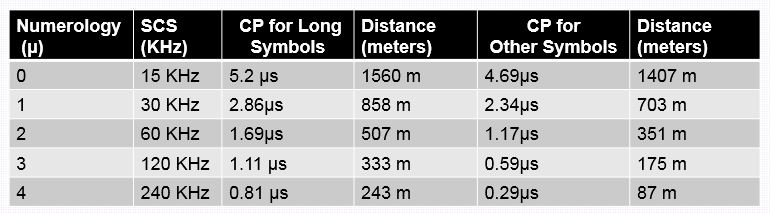Reference

• 3GPP References: TS 38.211 5G;. NR;. Physical channels and modulation

Related Post